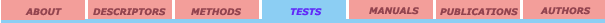External validation set Leave-One-Out crossvalidation Leave-Many-Out crossvalidation Randomization test

## Leave-One-Out crossvalidation

The simplest, and a commonly used method of crossvalidation in chemometrics is the “leave-one-out” method. The idea behind this method is to predict the property value for a compound from the data set, which is in turn predicted from the regression equation calculated from the data for all other compounds. For evaluation, predicted values can be used for PRESS, RMSPE, and squared correlation coefficient criteria (r2cv).

The method tends to include unnecessary components in the model, and has been provided  to be asymptotically incorrect. Furthermore, the method does not work well for data with strong clusterization,  and underestimates the true predictive error. 

References:

1. Eriksson, L.; Johansson, E.; Muller, M.; Wold, S. On the selection of the training set in environmental QSAR analysis when compounds are clustered J. Chemometrics 2000, 14, 599-616.

2. Stone M. An Asymptotic Equivalence of Choice of Model by Cross-Validation and Akaike’s Criterion  J. R. Stat. Soc., B 1977, 38, 44-47.
3. Martens, H.A.; Dardenne, P. Validation and verification of regression in small data sets 1998, 44, 99-121.University of Florida 2001. All rights reserved